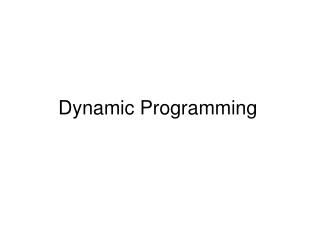DownloadDownload PresentationDynamic Programming

# Dynamic Programming

Download Presentation## Dynamic Programming

- - - - - - - - - - - - - - - - - - - - - - - - - - - E N D - - - - - - - - - - - - - - - - - - - - - - - - - - -
##### Presentation Transcript

1. Dynamic Programming

2. A typical infinite horizon problem (1) (2) (Intertemporal constraint.) (3) (Initial condition.) State xt Control ut

3. Value function Finite time horizon example

4. Hamilton-Jacobi-Bellman equation Solution Method (1) Backward induction (2) Optimality principle (HJB equation)

5. Optimality principle Relationship between value functions in adjacent periods Bellman equation)

6. Transversality condition

7. Solving dynamic programming problem using HJB equation • Assume a functional form of V(x) (which has free parameters • to be determined later). (2) Obtain the first order condition from (3) Use HJB equation to determine free parameters. (4) Ensure solutions satisfy initial and transversality conditions.

8. Variational Approach Euler equation as the optimality condition Choosing u(t) is equivalent to choosing x(t+1).

9. Derivation of the Euler equation First order condition Second order difference equation.

10. Transversality condition

11. Solving dynamic programming problem using variational method (1) Obtain the first order condition (Euler equation) (2) Solve for the general solutions to the Euler equation. (3) Use initial and transversality conditions to determine the unique solution.

12. Examples Value function (1) Bellman equation (2)

13. HJB approach (i) Postulate the form of the value function (ii) Obtain the FOC

14. (iii) Determine parameters (a) Obtain Riccardi equations using HJB Equating coefficients Solving Riccardi equations

15. Optimal policy and resulting dynamics (b) Boundary conditions Transversality Initial condition

16. Variational approach (i) Obtain the Euler equation Period t and t+1 payoffs Euler equation (ii) Solving the Euler equation

17. (iii) Use boundary conditions to get the unique solution Transversality Initial condition (iv) Final results